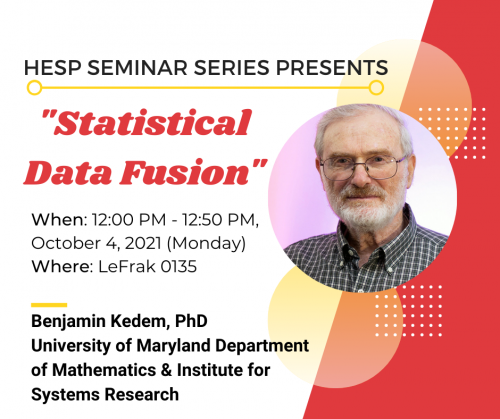Event Date and Time
-
Location
LEF0135
Speaker: Benjamin Kedem, PhD
Affiliation: University of Maryland Department of Mathematics & Institute for Systems Research
Title:  Statistical Data Fusion
Abstract: The density ratio model provides an inferential framework for semiparametric inference vis-a-vis fused data, such as meteorological satellite data fused with ground truth, fused data from several sensors, and fused case and control data. A concrete application where the density ratio model is used in connection with fused data is equi-distribution testing, this leading to a great generalization of the one-way ANOVA, obviating the normal assumption. Another application is time series prediction by predictive distributions. Yet another concrete example where the density ratio model is used is the estimation of small tail probabilities using numerous fusions
(could be millions) of real and computer generated data in what nowadays is called augmented reality. In this talk we shall:
• Review the density ratio model and some of its basic underpinnings.
• Discuss briefly a Bayesian extension applied to radar data.
• Discuss time series prediction by out of sample fusion.
• Argue that at times augmented reality is “better than real”, a case in point is the estimation of small tail probabilities.
Regarding the estimation of small tail probabilities, often, it is required to estimate the probability that a quantity such as mercury, lead, toxicity level, plutonium, temperature, rainfall, damage, wind speed, risk, etc., exceeds an unsafe high threshold. The probability in question is then very small. To estimate such a probability, we need information about large values of the quantity of interest. However, in many cases, the data only contain values far below the designated threshold, let alone exceedingly large values, which ostensibly renders the problem insolvable. It is shown that by repeated fusion of the data with externally generated random data, more information about small tail probabilities is obtained with the aid of certain new statistical functions. This provides short, yet reliable interval estimates based on moderately large samples. A comparison of the approach with a method from extreme values theory (Peaks over Threshold, or POT), using both artificial and real data, points to the merit of repeated out of sample fusion.